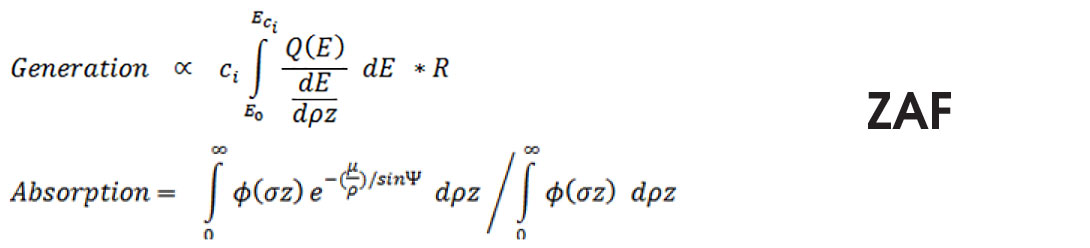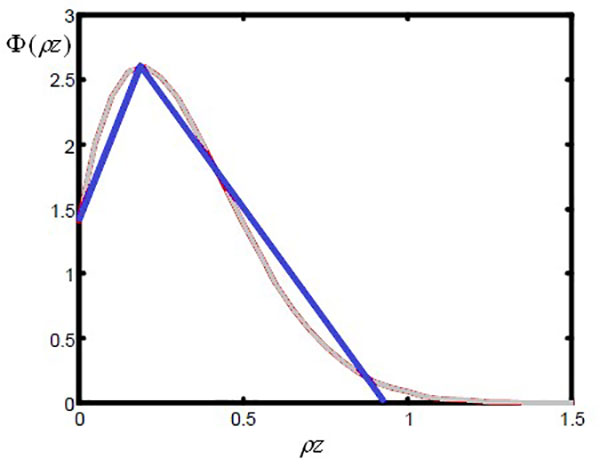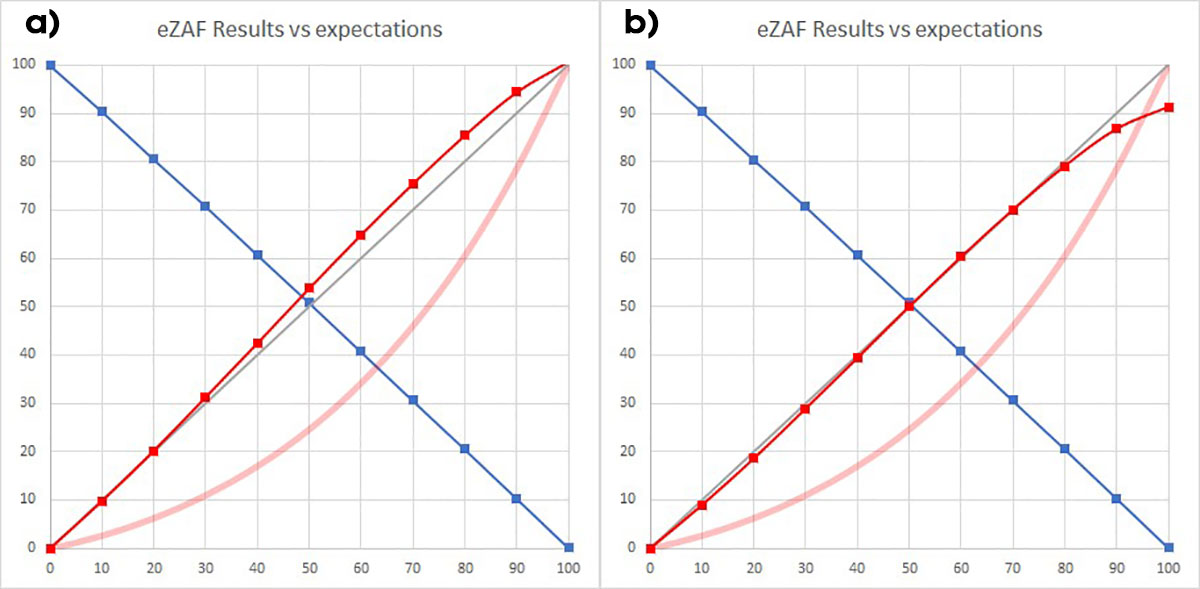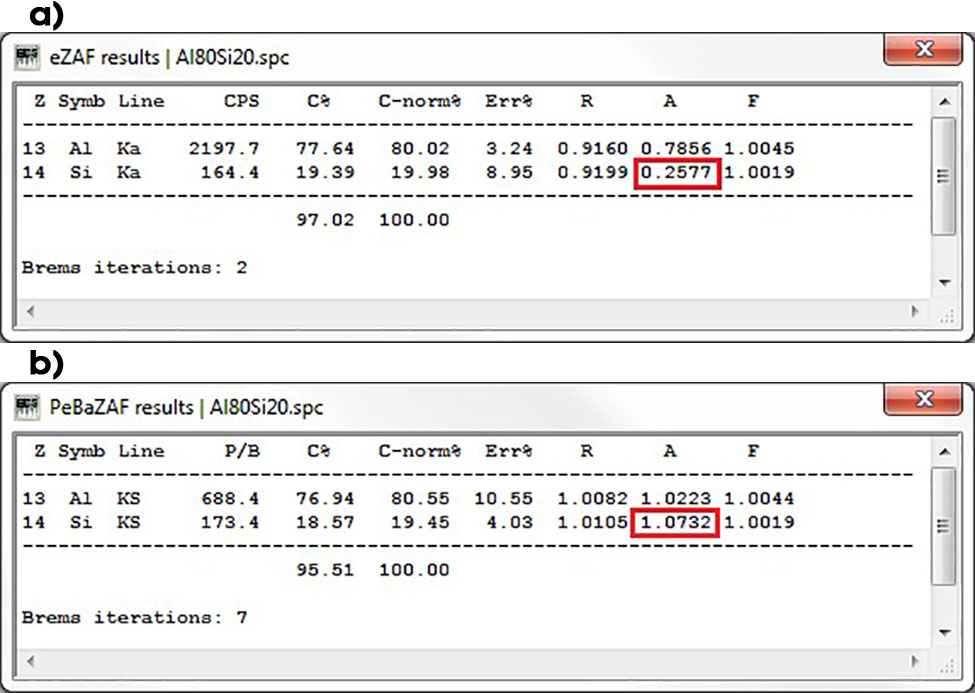Skip to content

# Ways to Improve the EDS Quantitative Results Accuracy: eZAF MACC Database and Correction Avoidance

### Introduction

It was shown with the Al/Si binary specimen example in the September 2021 issue of EDAX Insight that the results are within about 20% relative deviations (with the Si concentration <30%). Applying the SCC-based empirical database can improve accuracy in limited concentration ranges for dedicated applications. But it can amplify the deviations if the sample composition falls outside of the dedicated concentration range. The SCC factors are used to adjust the Generation of X-rays for individual elements and different excitation energies. SCC use is limited to handling the non-linear effects. For this reason, a second eZAF-dedicated database was introduced. It is two-dimensional and is focused to interelement dependencies, the Absorption.

### Results and Discussion

How is the Absorption modeled with eZAF? There are, in principle, two ways of considering the Generation and Absorption: The classical ZAF and the already more than two decades old Φ(ρz):

ZAF-approach:Equations 1 & 2.

The Generation equation calculates the electron shell excitation, the integral from primary electron E0 over all electron energies until the shell critical excitation energy EC is reached, considering the energy-dependent cross-section Q(E) and energy loss of electrons into specimen depth. It is an integral, complete depth distribution that considers the absorption of the X-rays which is different with each depth. A backscatter loss R is also considered. This term calculates the total X-ray generation by the electrons. The Absorption is decoupled into an extra equation within the ZAF model. It is an integral “depth distribution” of generated X-rays, over the depth into the specimen, considering absorption paths and Mass Absorption Coefficients (MACs), the µ/ρ. The total Absorption is then normalized to the same integral but without the absorption effect. The relative Absorption, the fraction of total generated X-rays, is calculated in this way. The quotient is then the same as the Generation term. The non-linear nature of the absorption processes is visible. The classical ZAF approach requires a formula for Φ(ρz) which can be integrated analytically.

Φ(ρz)-approach:Equation 3.

The younger Φ(ρz)-approach was originally developed based on the ability to measure depth distributions and the curves were improved and extended by Monte-Carlo calculations. There is no longer a requirement or limitation of the curves, they are numerically integrated. But in principle, the ZAF- approach can be solved with numerical integration of the equations. Therefore, the depth-distribution model is the only differentiator, not the kind of notation, comparing the ZAF-model vs. Φ(ρz)-model.Figure 1. The blue curve is the eZAF model using depth distribution, which is based on Love/Scott  with modification for tilted specimens . It was based on the Monte Carlo (MC) method calculations. The gray line is a comparison of a typical measurement or MC-calculated Φ(ρz).

There is no principle advantage of a Φ(ρz)-approach vs. ZAF. Moreover, following a statement by Heinrich, the ZAF is better suited to keep the different effects separated to improve each of the best, to get a better combined approach, and the best full correction model .

The foundation of Φ(ρz) is based on measured depth-distributions using a specified MAC database. Therefore, the Φ(ρz) includes a measured database fundamentally in genes. It is not a first principle-based standardless approach.

Several investigations have shown that the different depth distribution models, including the triangular Love/Scott model, do produce comparable results.

The most influencing parameters are the MACs, meaning the µ/ρ-values in model equations, which are atomic data that depend on X-ray energy and the transmitting material. Therefore, the Quant calculations do use one value for each element in the ID-list, the selected line-energy for all element- materials. A mean µ/, which is calculated by composition, is used. A calculated database is required with all possible elements alpha-lines, K-, L-, and M-radiation, which are possibly absorbed into any potential element. These required values are approximately 3 x 100 x 100.Figure 2. The used MAC values for an eZAF Quant evaluation with seven elements that are assumed in the specimen.

The idea is to apply a two-dimensional database at the MAC use level in the absorption model, to adjust the A-correction empirically. It is required for element combinations where the absorption effect is huge in element interdependencies.

Net-counts = X-ray Generation * SCC * Absorption (MACC) * Fluorescence * Efficiency (E) * ECF

It is applied in the eZAF model where the MACs are used inside the exponential functions, and it is named Mass Absorption Coefficients Correction (MACC). It is possible to adjust the element-lines energy absorptions in all possible absorber elements. It is adjusting and influencing the Quant evaluation only if the dedicated element and absorption constellation are really in the specimen. This does not influence all other evaluation cases, e.g., the pure element Si quantitative evaluation is not affected by the MACC for Si in Al, just because no Al is really in the specimen for a pure Si.

So, we have followed Heinrich’s recommendation with separated Generation database improvement with SCC and have decoupled the Absorption improvement ability with MACC.

The results in Figure 3a were obtained by optimizing the eZAF Absorption correction with the modified MAC with the MACC database. The improved results with the maximum number of deviations are still only about 8% relative (Figure 3a) over the entire composition area. The original results without using the database were about 20% maximum relative deviations, and it was only possible to improve them with SCC factors for a limited composition area to about 10% (for “Customized Standardless”). But this was with the disadvantage outside the adjusted/ dedicated composition area; the results got runaways up to 30% relatively.Figure 3. a) Calculated concentration results by eZAF for a binary Al/Si example specimen (blue Al; red Si) over the Si nominal concentration, all in % units, an adapted MACC database was used for Si-K X-rays in Al. The broad light-red line is the Si net-count raw data curve, arbitrary units, not yet ZAF corrected. b) The same result, but in addition to the MACC, the SCC was adapted for a 50%/50% sample.

Therefore, the one value MACC has provided is a huge improvement over the entire composition range of the pattern binary sample.

We can also adjust the SCC, Figure 3b, applied to a 50%/50% spectrum. The curve is only visibly shifting by a factor, in principle, the curve shape is not bent any further. And this SCC adaption does not improve all result deviations.

The MACC database is not dedicated to a change- access for the software operator. This is adjusting the non-linear part of eZAF model. If it is not properly adjusted, it can have lasting effects for the overall analytical performance in other element combinations. A skilled and experienced person is required to adapt the values in the database for step- by-step accuracy improvement in the future.

The core of the eZAF Quant accuracy questions are the X-ray excitation and non-linear interelement matrix-effects. These must be considered for use with theory/models (and depend on assumptions about the other elements, weight fractions).

• The SCC database addresses the X-ray generation.
• Separate from this, the MACC database addresses the non-linear interelement effects, well separated from excitation.

The SCC and MACC databases are useful for adjusting the models and processes to get better results. This improves the correction models by empirical results but does not reduce big correction needs.

Another strategy is to simply avoid the huge corrections. Smaller corrections of raw measured data reduce the model and atomic data uncertainty influences the results. Figure 4a shows the corrections applied with an 80%Al/20%Si example with eZAF, with the MACC improvement already considered. The A-factor for Si is 0.2577, which means about 74% of all generated X-rays are absorbed in the specimen material. Figure 4b presents the correction need of raw measured data is one order of magnitude less.Figure 4. a) Evaluation result with eZAF, Si-K absorption correction is within about 74%. b) Evaluation result with PeBaZAF, Si absorption correction is about 7.3%. This means the P/B based PeBaZAF correction requirement is one order of magnitude less.

This is an important property and advantage with PeBaZAF correction. It is due to the already discussed linearized P/B curve raw measurement signal (September 2021 issue of EDAX Insight Figure 1).

There are huge differences in correction needs. So, it is no surprise that the net-count-based quantification requires measured databases if good overall standardless accuracy is wanted. It is not possible to apply ZAF only based on fundamental parameter physics. The P/B model-based PeBaZAF makes it easier because the uncertainties in models and parameters do not affect the results to the same extent . The PeBaZAF requires less correction , independently from specimen absorption and MACs which have well-known huge uncertainties (Z ≥ 11). The reasons are because the characteristic X-ray Generation and especially the Absorption have similar effects when compared to bremsstrahlung for same the X-ray energy. A MACC database is not required.

Is it also possible to reduce correction needs significantly with eZAF, for further improvements of Quant, to get rid of the huge absorption correction needed for the example?

Yes, it is. One needs to measure standards or use already measured standards libraries… See the final third article about the topic.

### References

 Love F, Scott V D (1978) Journal of Physics D 11, 1369
 Sewell D A, Love F, Scott V D (1987) Journal of Physics D 20, 1567
 Heinrich K F J (1995) “An Evaluation of Quantitative Electron Probe Methods” in X-ray Spectrometry in Electron Beam Instruments, D B Williams, J I Goldstein, D E Newbury, Eds. (Plenum, New York) 305-367
 Eggert F (2018) “The P/B-Method, About 50 Years a Hidden Champion” Microscopy and Microanalysis 24, 734-735
 Eggert F (2019) “Complementary Standardless Quantitative Methods with EDS” Microscopy and Microanalysis 25, 560-561